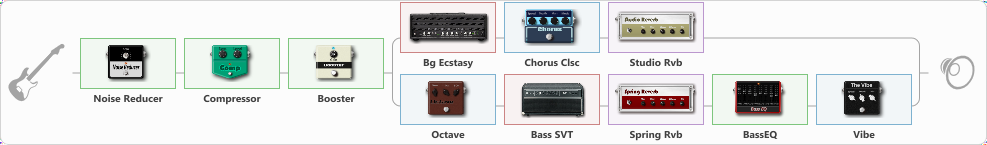# Bass Dual Vibe

Discussion in 'ToneLib-GFX presets' started by PaulTreff, Apr 26, 2020.

1. Bass Dual Vibe
Preset name: Bass Dual Vibe

Effects chain:Effect: "Noise Reducer" (Dynamics / Filter), active - "yes"
{

"Sens" = 66
"Mode" = Hard
}

Effect: "Compressor" (Dynamics / Filter), active - "yes"
{

"Sense" = 21
"Level" = 50
}

Effect: "Booster" (Dynamics / Filter), active - "yes"
{

"Gain" = 25
}

Effect: "Splitter" (Dynamics / Filter), active - "yes"
{

"A-Bypass" = Off
"A-Pan" = 0
"A-Level" = 55
"B-Bypass" = Off
"B-Pan" = 0
"B-Level" = 55
'A' branch:
{

Effect: "Bg Ecstasy" (Amp simulators), active - "yes"
{

"Gain" = 50
"Bass" = 50
"Middle" = 50
"Treble" = 50
"Presence" = 50
"Master" = 50
"Level (dB)" = 0
}
Effect: "Chorus Clsc" (Modulation / Sfx), active - "yes"
{

"Speed" = 3.5
"Depth" = 58
"Center" = 1.0
"Mode" = Stereo
}
Effect: "Studio Rvb" (Reverb), active - "yes"
{

"Time" = 7.4
"PreDelay" = 32
"LoDamp" = 0
"HiDamp" = 12
"Mix" = 58
}
}
'B' branch:
{

Effect: "Octave" (Modulation / Sfx), active - "yes"
{

"Direct" = 36
"-1 Oct" = 12
"-2 Oct" = 0
}
Effect: "Bass SVT" (Amp simulators), active - "yes"
{

"Bass Ctrl" = Off
"Midrange" = 200 Hz
"Ultra Hi" = Off
"Bass" = 3
"Middle" = -5
"Treble" = -3
"Volume" = 58
"Level (dB)" = 1
}
Effect: "Spring Rvb" (Reverb), active - "yes"
{

"Time" = 7.0
"PreDelay" = 0
"LoDamp" = 16
"HiDamp" = 26
"Mix" = 42
}
Effect: "BassEQ" (Dynamics / Filter), active - "yes"
{

"50 Hz" = 4
"120 Hz" = 4
"400 Hz" = 5
"500 Hz" = 6
"800 Hz" = 3
"4.5 kHz" = -5
"10 kHz" = 0
"Level (dB)" = 0
}
Effect: "Vibe" (Modulation / Sfx), active - "yes"
{

"Speed" = 5.0
"Depth" = 50
"Mix" = 50
}
}
}

Note: You will need to download and install the ToneLib-GFX software to use the preset.

#### Attached Files:

• ###### Bass_Dual_Vibe.tlgfx
File size:
1.1 KB
Views:
3,007
Last edited: Apr 26, 2020